# RL.ppt

31 de May de 2023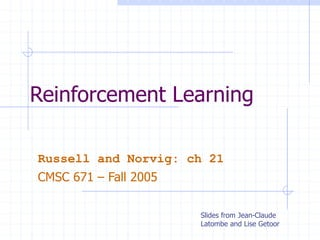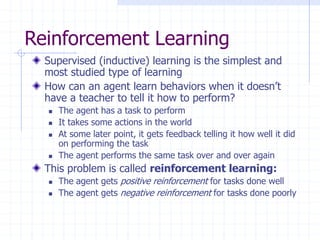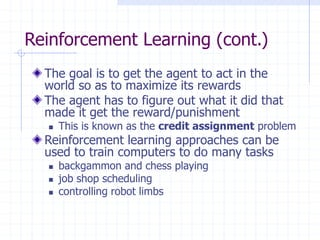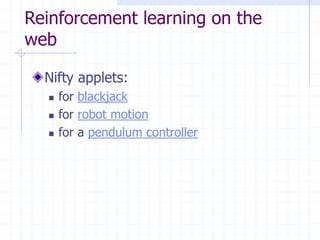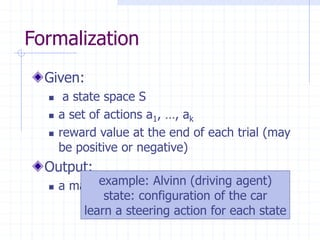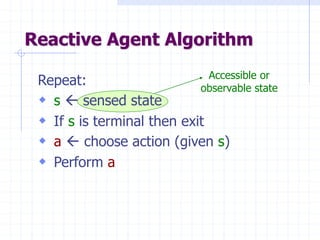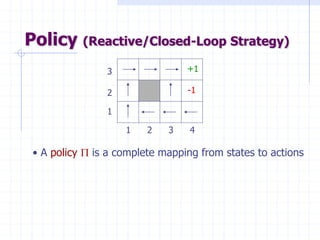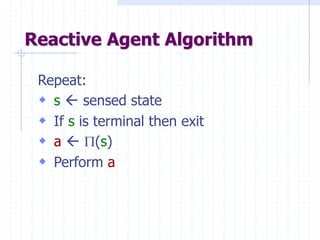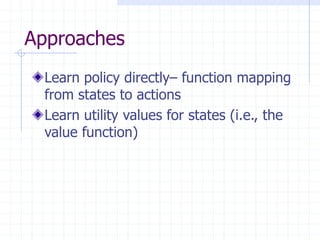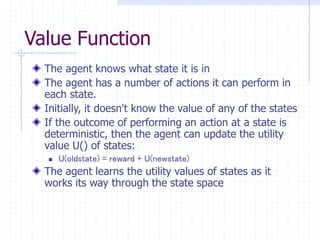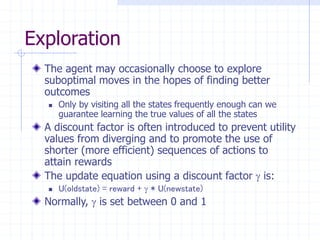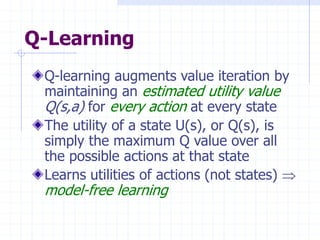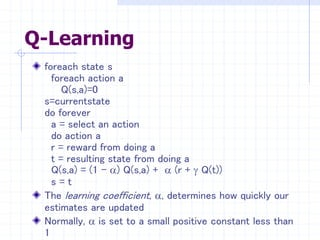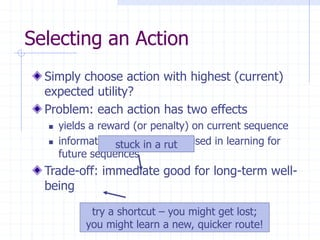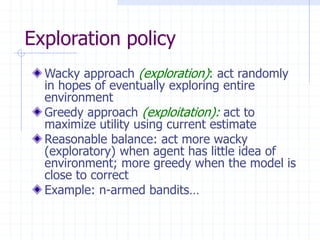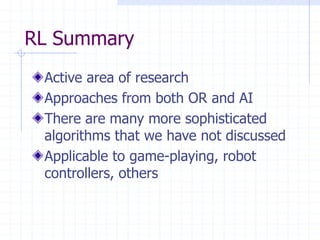1 de 16

### RL.ppt

• 1. Reinforcement Learning Russell and Norvig: ch 21 CMSC 671 – Fall 2005 Slides from Jean-Claude Latombe and Lise Getoor
• 2. Reinforcement Learning Supervised (inductive) learning is the simplest and most studied type of learning How can an agent learn behaviors when it doesn’t have a teacher to tell it how to perform?  The agent has a task to perform  It takes some actions in the world  At some later point, it gets feedback telling it how well it did on performing the task  The agent performs the same task over and over again This problem is called reinforcement learning:  The agent gets positive reinforcement for tasks done well  The agent gets negative reinforcement for tasks done poorly
• 3. Reinforcement Learning (cont.) The goal is to get the agent to act in the world so as to maximize its rewards The agent has to figure out what it did that made it get the reward/punishment  This is known as the credit assignment problem Reinforcement learning approaches can be used to train computers to do many tasks  backgammon and chess playing  job shop scheduling  controlling robot limbs
• 4. Reinforcement learning on the web Nifty applets:  for blackjack  for robot motion  for a pendulum controller
• 5. Formalization Given:  a state space S  a set of actions a1, …, ak  reward value at the end of each trial (may be positive or negative) Output:  a mapping from states to actions example: Alvinn (driving agent) state: configuration of the car learn a steering action for each state
• 6. Accessible or observable state Repeat:  s  sensed state  If s is terminal then exit  a  choose action (given s)  Perform a Reactive Agent Algorithm
• 7. Policy (Reactive/Closed-Loop Strategy) • A policy P is a complete mapping from states to actions -1 +1 2 3 1 4 3 2 1
• 8. Repeat:  s  sensed state  If s is terminal then exit  a  P(s)  Perform a Reactive Agent Algorithm
• 9. Approaches Learn policy directly– function mapping from states to actions Learn utility values for states (i.e., the value function)
• 10. Value Function The agent knows what state it is in The agent has a number of actions it can perform in each state. Initially, it doesn't know the value of any of the states If the outcome of performing an action at a state is deterministic, then the agent can update the utility value U() of states:  U(oldstate) = reward + U(newstate) The agent learns the utility values of states as it works its way through the state space
• 11. Exploration The agent may occasionally choose to explore suboptimal moves in the hopes of finding better outcomes  Only by visiting all the states frequently enough can we guarantee learning the true values of all the states A discount factor is often introduced to prevent utility values from diverging and to promote the use of shorter (more efficient) sequences of actions to attain rewards The update equation using a discount factor  is:  U(oldstate) = reward +  * U(newstate) Normally,  is set between 0 and 1
• 12. Q-Learning Q-learning augments value iteration by maintaining an estimated utility value Q(s,a) for every action at every state The utility of a state U(s), or Q(s), is simply the maximum Q value over all the possible actions at that state Learns utilities of actions (not states)  model-free learning
• 13. Q-Learning foreach state s foreach action a Q(s,a)=0 s=currentstate do forever a = select an action do action a r = reward from doing a t = resulting state from doing a Q(s,a) = (1 – ) Q(s,a) +  (r +  Q(t)) s = t The learning coefficient, , determines how quickly our estimates are updated Normally,  is set to a small positive constant less than 1
• 14. Selecting an Action Simply choose action with highest (current) expected utility? Problem: each action has two effects  yields a reward (or penalty) on current sequence  information is received and used in learning for future sequences Trade-off: immediate good for long-term well- being stuck in a rut try a shortcut – you might get lost; you might learn a new, quicker route!
• 15. Exploration policy Wacky approach (exploration): act randomly in hopes of eventually exploring entire environment Greedy approach (exploitation): act to maximize utility using current estimate Reasonable balance: act more wacky (exploratory) when agent has little idea of environment; more greedy when the model is close to correct Example: n-armed bandits…
• 16. RL Summary Active area of research Approaches from both OR and AI There are many more sophisticated algorithms that we have not discussed Applicable to game-playing, robot controllers, others